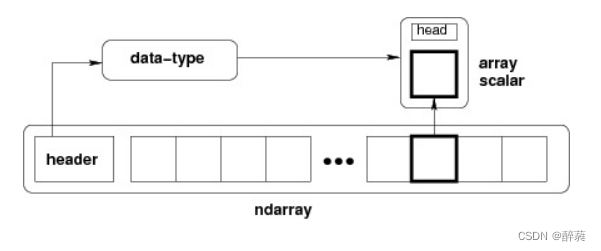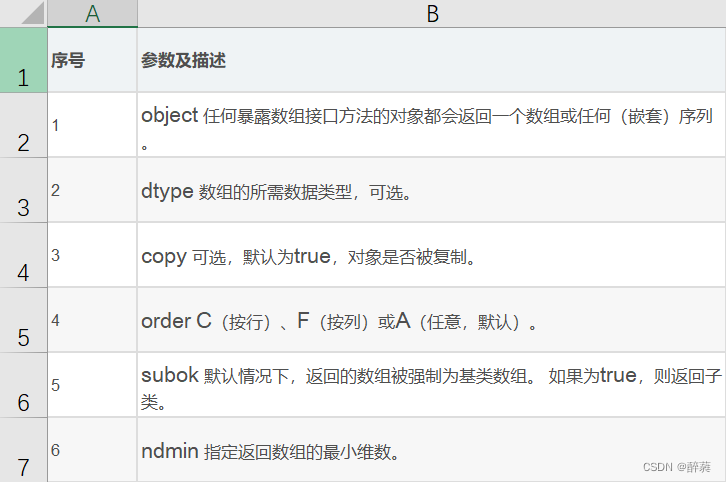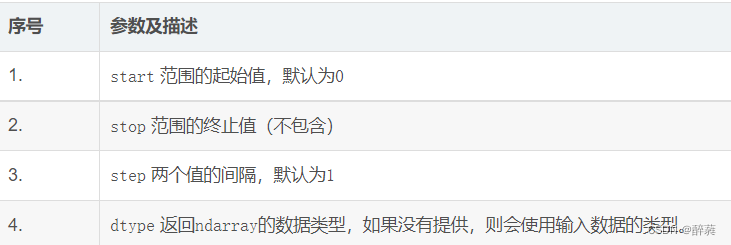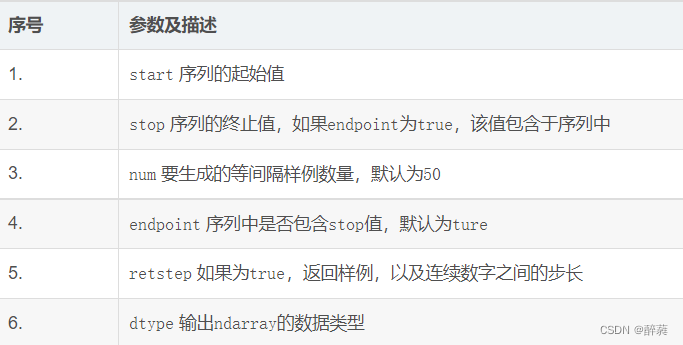### Python之numpy函数​

# 学习日记

1、numpy简介

2、numpy安装

1、概述

2、ndarray特点

3、内部结构

1、ndarray.shape

2、ndarray.ndim（返回数组的维数）

3、numpy.itemsize（返回数组中每个元素的字节单位长度）

4、numpy.arange（返回ndarray对象，包含给定范围内的等间隔值）

5、numpy.linspace

​六、创建数组

1、通过列表创建一维数组

2、通过列表创建二维数组

3、创建全为0的二维数组

4、创建全为1的三维数组

5、full函数创建任意大小的数组并填充任意数字

6、创建一维等差数组

7、创建二维等差数组

8、创建单位矩阵（二维数组）

9、创建二维随机数组

10、创建二维随机整数数组（数值小于 6）

七、数组运算

1、和

2、mean

3、argsort

4、矩阵乘法

5、矩阵求逆

## 一、numpy概述

### 1、numpy简介

NumPy是一个功能强大的Python库，主要用于对多维数组执行计算。NumPy提供了大量的库函数和操作，可以帮助程序员轻松地进行数值计算。这类数值计算广泛用于机器学习模型、图像处理、数学任务等方面。

### 2、numpy安装

``````pip install numpy
``````

## 二、 Ndarray 对象

### 1、概述

NumPy 中定义的最重要的对象是称为 `ndarray` 的 N 维数组类型。  它是一系列同类型数据的集合，以 0 下标为开始进行集合中元素的索引。

`        ndarray`中的每个元素在内存中使用相同大小的块。 `ndarray`中的每个元素是数据类型对象的对象（称为 `dtype`）。从`ndarray`对象提取的任何元素（通过切片）由一个数组标量类型的 Python 对象表示。 下图显示了`ndarray`，数据类型对象（`dtype`）和数组标量类型之间的关系。

### 2、ndarray特点

ndarray 对象是用于存放同类型元素的多维数组。

ndarray 中的每个元素在内存中都有相同存储大小的区域。

### 3、内部结构``#基本的Ndarray 是使用 NumPy 中的数组函数创建的。numpy.array ``

``````numpy.array(object, dtype = None, copy = True, order = None, subok = False, ndmin = 0)
``````## 三、数据类型对象 (`dtype`)

`dtype`可由语法构造

``numpy.dtype(object, align, copy)``
• `Object`：被转换为数据类型的对象。

• `Align`：如果为`true`，则向字段添加间隔，使其类似 C 的结构体。

• `Copy` ? 生成`dtype`对象的新副本，如果为`flase`，结果是内建数据类型对象的引用。

`` # 使用数组标量类型  import numpy as np dt = np.dtype(np.int32)  print dt``

结果

``````int32
``````

## 四、NumPy 创建数组

numpy.empty 方法用来创建一个指定形状（shape）、数据类型（dtype）且未初始化的数组

``numpy.empty(shape, dtype = float, order = 'C')``

numpy.zeros 创建指定大小的数组，数组元素以 0 来填充

``````numpy.zeros(shape, dtype = float, order = 'C')
``````

numpy.ones 创建指定形状的数组，数组元素以 1 来填充

``numpy.ones(shape, dtype = None, order = 'C')``

## 五、数组属性

### 1、`ndarray.shape`

``import numpy as np a = np.array([[1,2,3],[4,5,6]])  print a.shape``

``````(2, 3)
``````
`` # 调整数组大小  import numpy as np  a = np.array([[1,2,3],[4,5,6]]) a.shape =  (3,2)  print a ``

``[[1, 2]  [3, 4]  [5, 6]]``

### 2、`ndarray.ndim（`返回数组的维数`）`

`` # 等间隔数字的数组  import numpy as np a = np.arange(24)  print a``

``[0 1  2  3  4  5  6  7  8  9  10  11  12  13  14  15  16 17 18 19 20 21 22 23] ``
`` # 一维数组  import numpy as np a = np.arange(24) a.ndim # 现在调整其大小b = a.reshape(2,4,3)  print b # b 现在拥有三个维度``

``[[[ 0,  1,  2]   [ 3,  4,  5]   [ 6,  7,  8]   [ 9, 10, 11]]    [[12, 13, 14]    [15, 16, 17]   [18, 19, 20]    [21, 22, 23]]] ``

### 3、`numpy.itemsize（`返回数组中每个元素的字节单位长度`）`

`` # 数组的 dtype 为 int8（一个字节）  import numpy as np x = np.array([1,2,3,4,5], dtype = np.int8)  print x.itemsize``

``1``

### 4、`numpy.arange（`返回`ndarray`对象，包含给定范围内的等间隔值）

`` numpy.arange(start, stop, step, dtype)```` import numpy as npx = np.arange(5)  print x``

结果

``[0  1  2  3  4]``
`` import numpy as np# 设置 dtypex = np.arange(5, dtype =  float)  print x``

``[0.  1.  2.  3.  4.]``
`` # 设置了起始值和终止值参数  import numpy as npx = np.arange(10,20,2)  print x``

``[10  12  14  16  18]``

### 5、`numpy.linspace`

`````` numpy.linspace(start, stop, num, endpoint, retstep, dtype)
``````## ​六、创建数组

### 1、通过列表创建一维数组

``np.array([1, 2, 3])``

array([1, 2, 3])

### 2、通过列表创建二维数组

``np.array([(1, 2, 3), (4, 5, 6)])``

array([[1, 2, 3], [4, 5, 6]])

### 3、创建全为0的二维数组

``np.zeros((3, 4))``

array([[0., 0., 0., 0.],
[0., 0., 0., 0.],
[0., 0., 0., 0.]])

### 4、创建全为1的三维数组

``np.ones((2, 3, 4))``

array([[[1., 1., 1., 1.],
[1., 1., 1., 1.],
[1., 1., 1., 1.]],
[[1., 1., 1., 1.],
[1., 1., 1., 1.],
[1., 1., 1., 1.]]])

### 5、full函数创建任意大小的数组并填充任意数字

``np.full((3, 4), 2)``

array([[2, 2, 2, 2],
[2, 2, 2, 2],
[2, 2, 2, 2]])

### 6、创建一维等差数组

``np.arange(5)``

array([0, 1, 2, 3, 4])

### 7、创建二维等差数组

``np.arange(6).reshape(2, 3)``

array([[0, 1, 2],
[3, 4, 5]])

### 8、创建单位矩阵（二维数组）

``np.eye(3)``

array([[1., 0., 0.],
[0., 1., 0.],
[0., 0., 1.]])

### 9、创建二维随机数组

``np.random.rand(2, 3)``

### 10、创建二维随机整数数组（数值小于 6）

``````np.random.randint(6, size = (2, 3))
``````

## 七、数组运算

a = np.array([10, 20, 30, 40, 50])
b = np.arange(1, 6)

### 1、和

``a = np.array([[1, 2],              [3, 4]])np.sum(a)  np.sum(a, axis=0) # axis=0 表示对每一列求和  np.sum(a, axis=1) # axis=0 表示对每一行求和``

### 2、mean

``np.mean(a) # 求取平均值``

### 3、argsort

``a = np.array([[3, 6, 4, 11],              [5, 10, 1, 3]])a.argsort() # 将元素按照行从小到大排序，返回对应位置元素的下标``

``array([[0, 2, 1, 3],   [2, 3, 0, 1]], dtype=int64)``

### 4、矩阵乘法

``````np.mat(A) * np.mat(B)
``````

### 5、矩阵求逆

``````np.linalg.inv(A)
``````